#### 期刊菜单

Distributed Resource Management Algorithm Based on LSTM Dueling Double DQN in V2V Communication
DOI: 10.12677/CSA.2023.131012, PDF, HTML, XML, 下载: 36  浏览: 97

Abstract: The World Health Organization (WHO) points out that in order to prevent traffic accidents that cause millions of deaths every year, the Vehicle-to-Everything (V2X) communication between vehicles and other vehicles, infrastructure or pedestrians is essential. V2 enables vehicles to broadcast safety information, including its position, speed and collision warning information, so as to reduce road accidents. With the progress of wireless communication technology and the development of intelligent driving technology, real-time delivery, low network cost and stable delivery of communication information have become the foundation and core of the rapid development of various intelligent transportation systems. However, in V2X communication, data transmission is usually carried out through a base station to allocate frequency and time resources to vehicles, and the stability of transmission cannot always be guaranteed. Moreover, the communication characteristics of wireless network and the mobility of vehicles in vehicle networking bring great challenges to vehicle networking communication services. In large-scale temporal data distribution scenarios, the traditional distributed service framework can’t only rely on base station services to meet the high-incidence heterogeneous network resource allocation and communication resource sharing, because the coverage of base stations is limited and vehicles are moving at high speed. In view of the above problems, this paper systematically studies the information service technology of vehicle networking from the perspective of service framework and algorithm design. The main achievements include the following aspects, the large-scale data distribution in V2X communication environment is studied. Firstly, a multi-agent temporal information service framework for distributed resource scheduling is proposed, and a distributed resource allocation model is established. On this basis, a distributed resource allocation algorithm for multi-agent reinforcement learning is proposed. Through the real-time interaction of vehicle inspection network, the reservation and reuse of wireless resources in dynamic environment are intelligently decided, which makes the resource selection adapt to the dynamic changes of the surrounding environment of vehicles, and significantly reduces the probability of group collision.

1. 引言

2. 研究现状

3. 系统模型和问题描述

3.1. 系统模型

$PR{R}_{t}^{i}\left({a}_{t}\right)=\frac{1}{{N}_{t}^{i}}{\sum }_{j=1}^{{N}_{t}^{i}}1\left\{{P}_{eer}\left({\gamma }_{t}^{i,j}\right) (1)

${\gamma }_{t}^{i,j}=\frac{P{|{H}_{t}^{i,j}|}^{2}}{{\sigma }^{2}+\sum P{|{H}_{t}^{k,j}|}^{2}},k\in \frac{{H}_{t}^{c}\left({a}_{t}\right)}{\left\{i\right\}}$ (2)

P函数是基于固定的调制编码方案(MCS)计算给定SINR下的误块率。如果P等于或小于一个介于0和1之间的随机数，则数据包将被成功解码。P是所有车辆固定的发射功率， ${\sigma }^{2}$ 是加性高斯白噪声的功率，N是由t时刻的动作决定的干扰数据包集合， $|{H}_{t}^{i,j}|$$|{H}_{t}^{k,j}|$ 分别是发送者i和接收者j之间以及发送者k和接收者j之间的信道增益。

3.2. 问题定义

$\begin{array}{l}\underset{\pi }{\mathrm{max}}\underset{i=1}{\overset{N}{\sum }}PR{R}_{t}^{i}\left({a}_{t}^{\pi }\right)\\ \text{subject}\text{\hspace{0.17em}}\text{to}\text{\hspace{0.17em}}\text{ }{a}_{t}^{i}\in \left(1,2,\cdots ,K\right)\end{array}$ (3)

4. 算法框架

4.1. D2DQN

4.2. 状态和奖励设计

1) 状态和动作空间

2) 奖励设计

${r}_{t}^{i}\left({a}_{t}^{i}|{s}_{t}^{i}\right)=\left\{\begin{array}{l}1\text{\hspace{0.17em}}\text{\hspace{0.17em}}\text{\hspace{0.17em}}\text{\hspace{0.17em}}\text{\hspace{0.17em}}\text{\hspace{0.17em}}\text{\hspace{0.17em}}\text{\hspace{0.17em}}\text{\hspace{0.17em}}\text{\hspace{0.17em}}\text{\hspace{0.17em}}\text{ }\text{ }{N}_{t}^{c}=1\\ 0\text{\hspace{0.17em}}\text{\hspace{0.17em}}\text{\hspace{0.17em}}\text{\hspace{0.17em}}\text{\hspace{0.17em}}\text{\hspace{0.17em}}\text{\hspace{0.17em}}\text{\hspace{0.17em}}\text{\hspace{0.17em}}\text{\hspace{0.17em}}\text{ }\text{\hspace{0.17em}}\text{if}\text{\hspace{0.17em}}dist\left(c\right)>{r}_{reuse}{N}_{t}^{c}=2\\ -{N}_{t}^{c}\text{\hspace{0.17em}}\text{\hspace{0.17em}}\text{\hspace{0.17em}}\text{\hspace{0.17em}}\text{\hspace{0.17em}}\text{\hspace{0.17em}}\text{\hspace{0.17em}}\text{else},{N}_{t}^{c}=2\\ -{N}_{t}^{c}\text{\hspace{0.17em}}\text{\hspace{0.17em}}\text{\hspace{0.17em}}\text{\hspace{0.17em}}\text{\hspace{0.17em}}\text{\hspace{0.17em}}\text{\hspace{0.17em}}{N}_{t}^{c}>2\end{array}$ (4)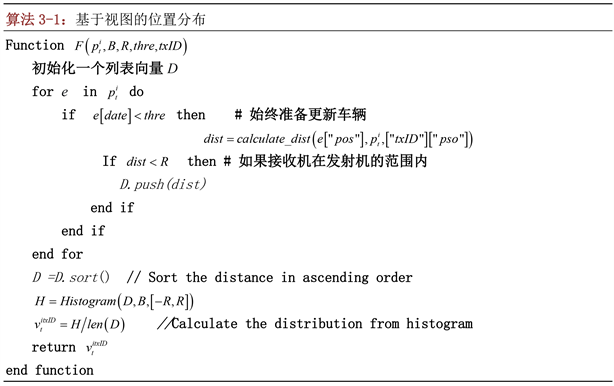Step 1：

Step 2：

Step 3：

Step 4：

Double DQN如果车辆选择和评估与同一网络的行动，由于过分估计使得算法的性能下降。因此，采用双Q学习实现动作选择与评价的解耦。$Q\left(s,a,\omega ,\alpha ,\beta \right)=V\left(s,\omega ,\alpha \right)+A\left(s,a,\omega ,\beta \right)$ (5)

5. 仿真结果

5.1. 训练参数设置

5.2. 训练效果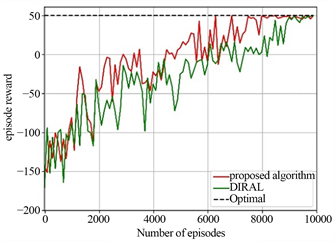Figure 1. 4 vehicles and 3 resource scenarios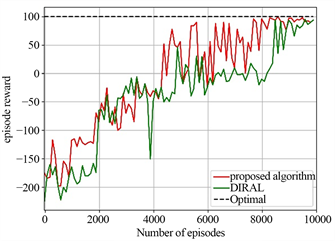Figure 2. 6 vehicles and 8 resource scenarios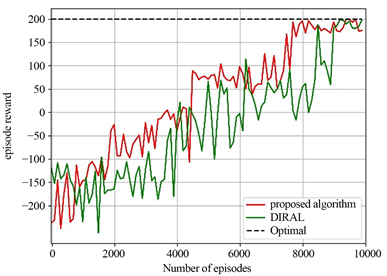Figure 3. 8 vehicles and 10 resource scenarios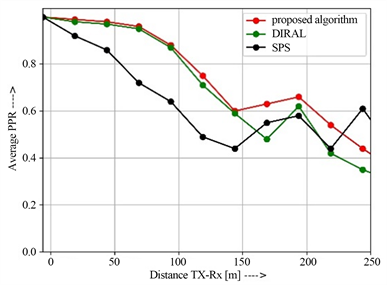Figure 4. RealNeS analysis

6. 结论